120 Volts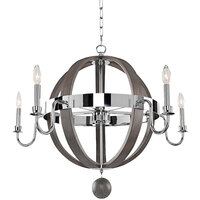\$1,016.00/Each120 Volts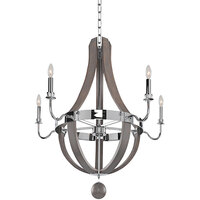\$1,180.00/Each120 Volts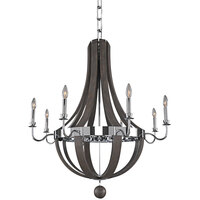\$1,534.00/Each120 Volts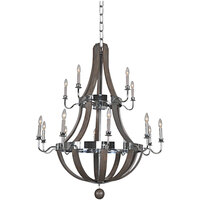\$2,358.00/Each120 Volts\$1,122.00/Each120 Volts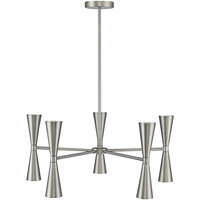\$1,234.00/Each120 Volts\$1,122.00/Each120 Volts\$1,684.00/Each120 Volts\$1,852.00/Each120 Volts\$1,684.00/Each120 Volts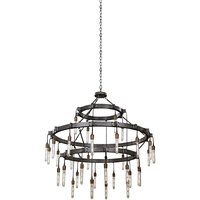\$2,330.00/Each120 Volts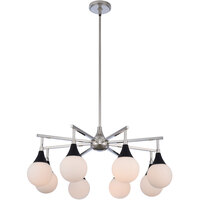\$528.00/Each120 Volts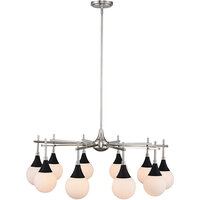\$634.00/Each120 Volts\$380.00/Each120 Volts\$498.00/Each120 Volts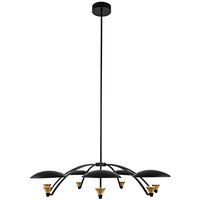\$698.00/Each120 Volts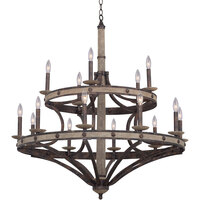\$2,548.00/Each120 Volts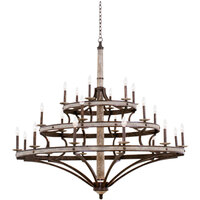\$7,078.00/Each120 Volts\$1,402.00/Each120 Volts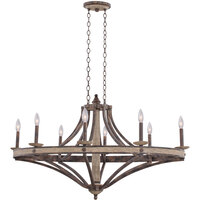\$1,784.00/Each120 Volts\$1,658.00/Each